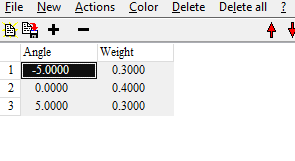# Angle of incidence distribution

Spectroscopic experiments can never realize single angle of incidence but have to work with a (continuous) distribution of angles. Although in most cases the assumption of a single angle of incidence is a very good approximation there are cases where we need to take into account more details in producing simulated spectra. One example is taking reflectance spectra of small spots with a microscope objective, using a large cone of incident radiation.

For a long time our software packages can compute spectra averaged for a set of incidence angles, each one defined by the value of the angle and a weight. We have now implemented new features to simplify work in this field.

If you have prepared a list of angles of incidence you can now connect these angles to the angle of incidence that you have defined for spectrum object which owns the list of angles. You can check the option as shown here:If you have activated this option you can use a set of angles with positive and negative values (centered around 0) as shown here:If the angle of the parent object is 50° the computation of the spectrum is done for the 3 angles 45°, 50° and 55°, with weights 0.3, 0.4 and 0.3, respectively. If you have declared the angle of the parent object to be a fit parameter the set of 3 angles is moved automatically when the value of the center angle changes during the fit. This helps to adjust the distribution of angles to match the experimental settings.

Finally, there is a new fit parameter called ‘Scaling factor of angle range’. This number scales the distance of the individual angles to the center angle: If the factor is 1.0 the original angles are used. If the factor is 0.1, for example, a value of 5° becomes 0.5°. Varying the factor between 0.1 and 2 in the example shown above, you can compute spectra for a distributions between -0.5° … 0.5° to -10° … 10°.We hope that this new flexibility helps to achieve better fitting results for spectra measured with microscopes or other systems with spectra features depending critically on the angle of incidence.